Start typing, then use the up and down arrows to select an option from the list.# Scientific Notation

Jules Bruno
235views
3
Now we're going to take a look at How exactly do we change standard notation into scientific notation? Now, the key is to look at the coefficient. Now, we're gonna say to convert a number into a scientific notation value, make sure the coefficient is equal to or greater than one, but less than 10. So what we learn the very beginning of scientific notation is the key to changing standard notation into scientific notation. So if we take a look here, we're gonna say increasing the coefficient makes the exponents value decrease. All right, so here we have a number of 0.145 We need to make sure that that coefficient, which we're going to make, has to fall between one and 10. Here is our decimal place. We're gonna have to move it. spaces so that we can get 1.45 which is a value between one and 10 now. We made the coefficient larger since we made the coefficient larger. That means the exponents has to become smaller, so the coefficient increased by five. So the exponents has to decrease by five because they're opposites of one another. Now, decreasing the coefficient value makes the exponents value increase. So here in this one, there is. We could think of there being a decimal point there, and we're gonna move it over enough spaces in order to make this coefficient a number between one and 10. So we're gonna move. One, aN:aN:000NaN spaces. So that becomes 1.13 to times 10. Now, we moved over eight spaces in order to make this smaller. So if the coefficient is decreasing by eight spaces, that means our exponents has to be increasing by eight spaces. So it'll be a positive eight here. So that would be the scientific notation value for this number here. Later on, we'll talk about things such a significant figures which will help us to even simplify this scientific notation, even mawr, because there's an awful lot of zeros here at the end. But for now, this would be the entire value that we place to show that we moved decimal place eight spaces over to make the coefficient smaller and thereby increasing the exploding by eight. Now that we've seen these examples move on to the practice question left at the bottom of the page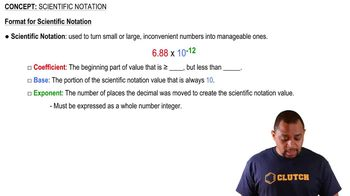01:41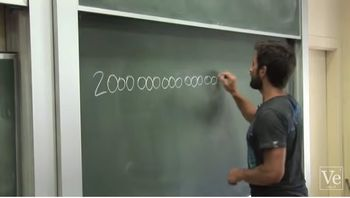05:13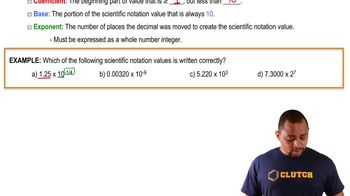01:53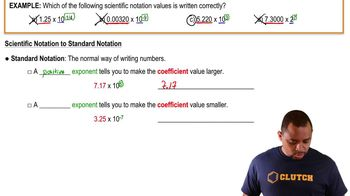02:42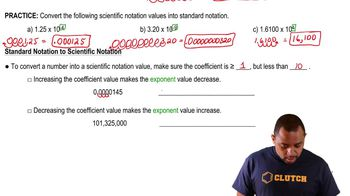02:50